## A motorcycle daredevil plans to ride up a 2.0-m-high, 20° ramp, sail across a 10-m-wide pool filled with hungry crocodiles, and land at grou

Question

A motorcycle daredevil plans to ride up a 2.0-m-high, 20° ramp, sail across a 10-m-wide pool filled with hungry crocodiles, and land at ground level on the other side. He has done this stunt many times and approaches it with confidence. Unfortunately, the motor-cycle engine dies just as he starts up the ramp. He is going 11 m/s at that instant, and the rolling friction of his rubber tires (coefficient 0.02) is not negligible. Does he survive, or does he become croco-dile food? Justify your answer by calculating the distance he travels through the air after leaving the end of the ramp

in progress 0
2 months 2021-07-31T23:03:14+00:00 1 Answers 2 views 0

He becomes a croco-dile food

Explanation:

From the question we are told that

The height is h = 2.0 m

The angle is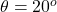The distance is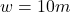The speed is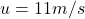The coefficient of static friction is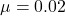At equilibrium the forces acting on the motorcycle are mathematically represented as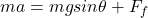where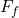is the frictional force mathematically represented as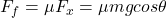where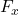is the horizontal component of the force

substituting into the equation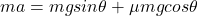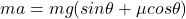making  a the subject of the formula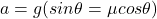substituting values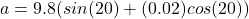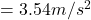Applying ” SOHCAHTOA” rule we mathematically evaluate that length of the ramp as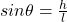makingthe subject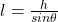substituting values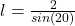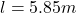Apply Newton equation of motion we can mathematically evaluate the  final velocity at the end of the ramp  as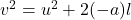The negative a means it is moving against gravity

substituting values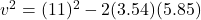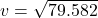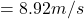The initial velocity at the beginning of the pool (end of ramp) is composed of two component which is

Initial velocity along the x-axis which is mathematically evaluated as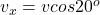substituting values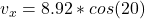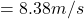Initial velocity along the y-axis which is mathematically evaluated as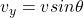substituting values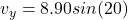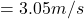Now the motion through the pool in the vertical direction can mathematically modeled as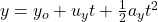where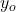is the initial height,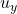is the initial velocity in the y-axis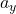is the  initial  acceleration in the y axis  with a constant value of (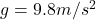)

at the y= 0 which is when the height above ground is zero

Substituting values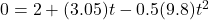The negative sign is because the acceleration is moving against the motion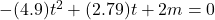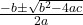substituting values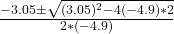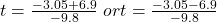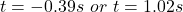since in this case time cannot be negative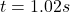At this time the position the  motorcycle along the x-axis is mathematically evaluated as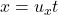x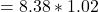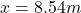So from this value we can see that the motorcycle would not cross the pool as the position is less that the length of  the pool